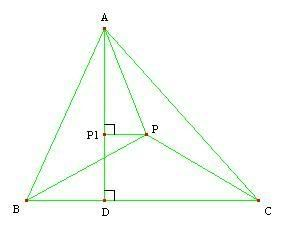## Sunday, March 26, 2006

### That's The Orthocenter! Topic: Geometry/Inequalities. Level: Olympiad.

Problem: (360 Problems For Mathematical Contests - 3.1.7) Let $a,b,c$ and $S$ be the side lengths and the area of triangle $ABC$. Prove that if $P$ is a point interior to triangle $ABC$ such that

$a \cdot PA+b \cdot PB+c \cdot PC = 4S$

then $P$ is the orthocenter of the triangle.Solution: Let $D, E, F$ be the feet of the altitudes from $A, B, C$ respectively. Denote $P_1, P_2, P_3$ the projections of $P$ onto $AD, BE, CF$, respectively. $D$ and $P_1$ are shown in the picture above.

We know $S = [PBC]+[PCA]+[PAB]$. And since the altitudes of these three smaller triangles from $P$ are equal in length to $P_1D = AD-AP_1$, $P_2E = BE-BP_2$, $P_3F = CF-CP_3$, respectively, we have

$[PBC] = \frac{1}{2}a(AD-AP_1)$

$[PCA] = \frac{1}{2}b(BE-BP_2)$

$[PAB] = \frac{1}{2}c(CF-CP_2)$.

So $S = \frac{1}{2}(a \cdot AD+ b \cdot BE+ c \cdot CF) - \frac{1}{2}(a \cdot AP_1 + b \cdot BP_2 + c \cdot CP_3)$, which rearranges to

$a \cdot AP_1+ b \cdot BP_2 + c\cdot CP_3 = a \cdot AD+ b \cdot BE+ c \cdot CF - 2S$. (1)

But since $\frac{1}{2} a \cdot AD = \frac{1}{2} b \cdot BE = \frac{1}{2} c \cdot CF = S$, we have

$a \cdot AD + b \cdot BE + c \cdot CF = 6S$. (2)

Substituting (2) into (1), we obtain

$a \cdot AP_1+ b \cdot BP_2 + c\cdot CP_3 = 6S-2S = 4S$.

However, we have $AP \ge AP_1$, $BP \ge BP_2$, and $CP \ge CP_3$ (since they are projections) so

$a \cdot AP + b \cdot BP + c \cdot CP \ge a \cdot AP_1+ b \cdot BP_2 + c\cdot CP_3 = 4S$.

But since the given condition implies equality, we know that $AP = AP_1$, $BP = BP_2$, and $CP = CP_3$, or that $P$ lies on all three altitudes it was projected on and hence is the orthocenter. QED.

--------------------

Comment: Many times geometric conditions often arise from inequalities that have a specific equality case. In this problem, we wanted to show something was the orthocenter so we related the given lengths to the altitudes, which worked.

--------------------

Practice Problem: (360 Problems For Mathematical Contests - 3.1.14) Let $I$ be the incenter of an acute triangle $ABC$. Prove that

$AI \cdot BC + BI \cdot CA + CI \cdot AB = 4S_{ABC}$

if and only if triangle $ABC$ is equilateral.